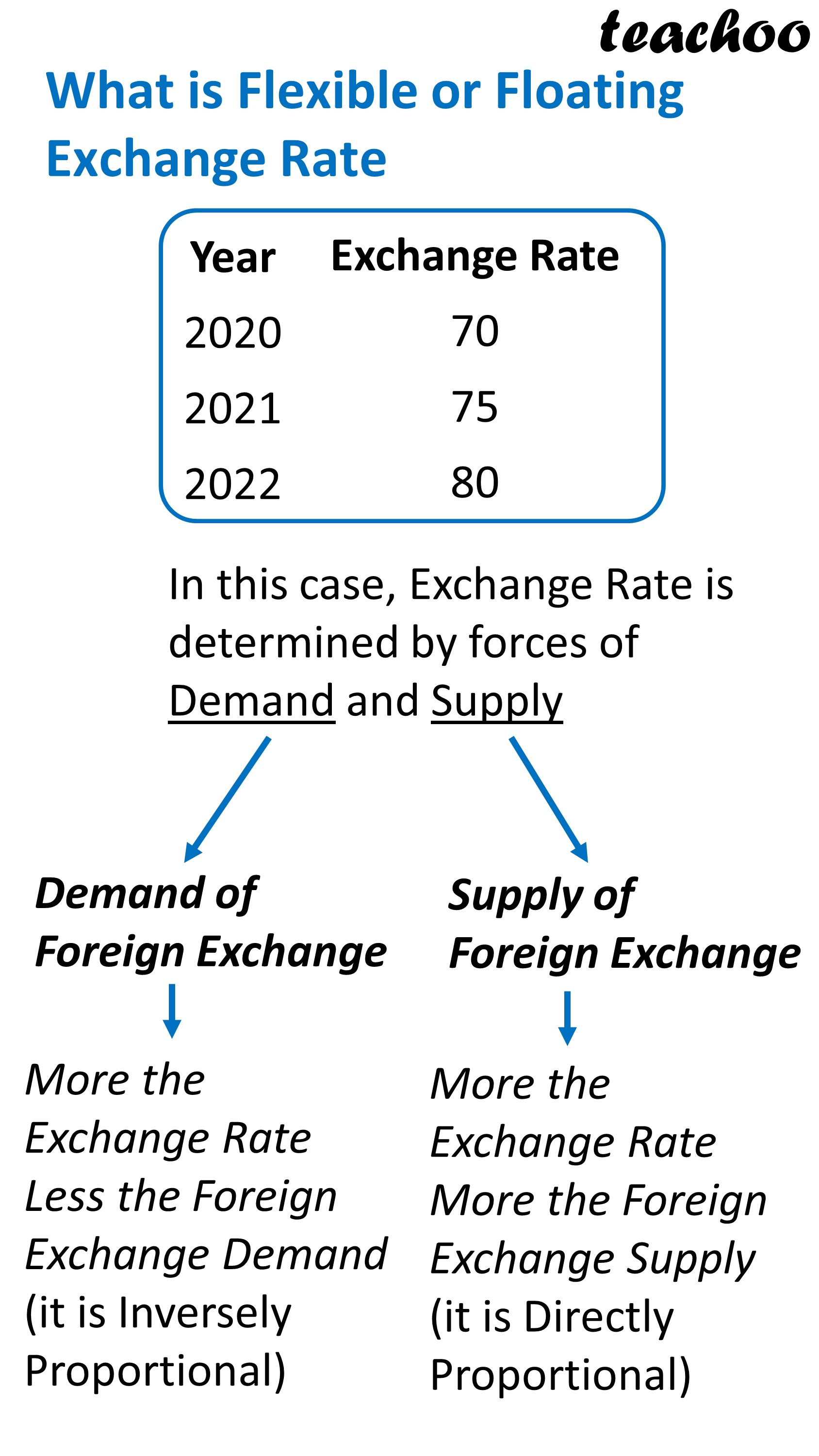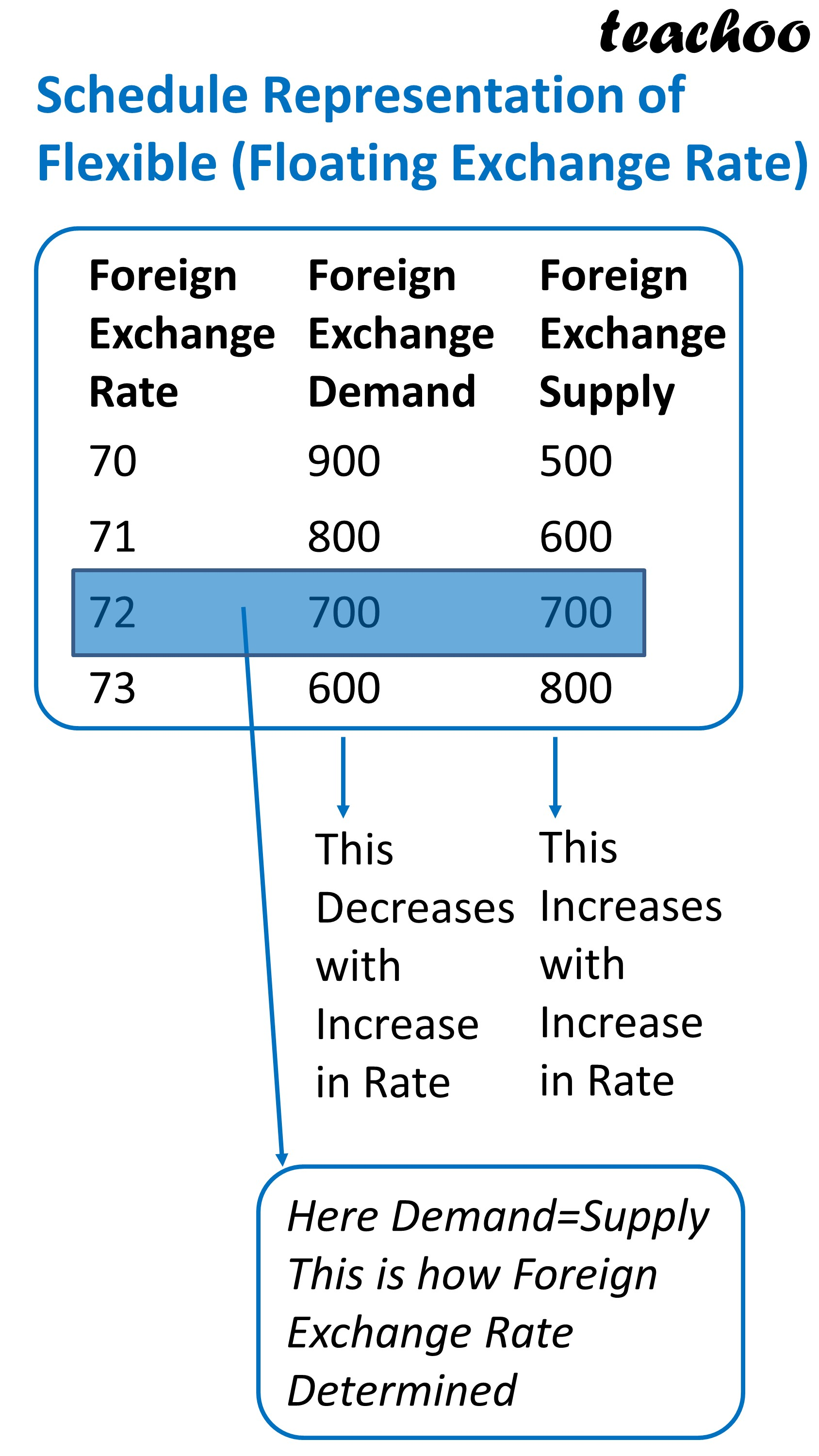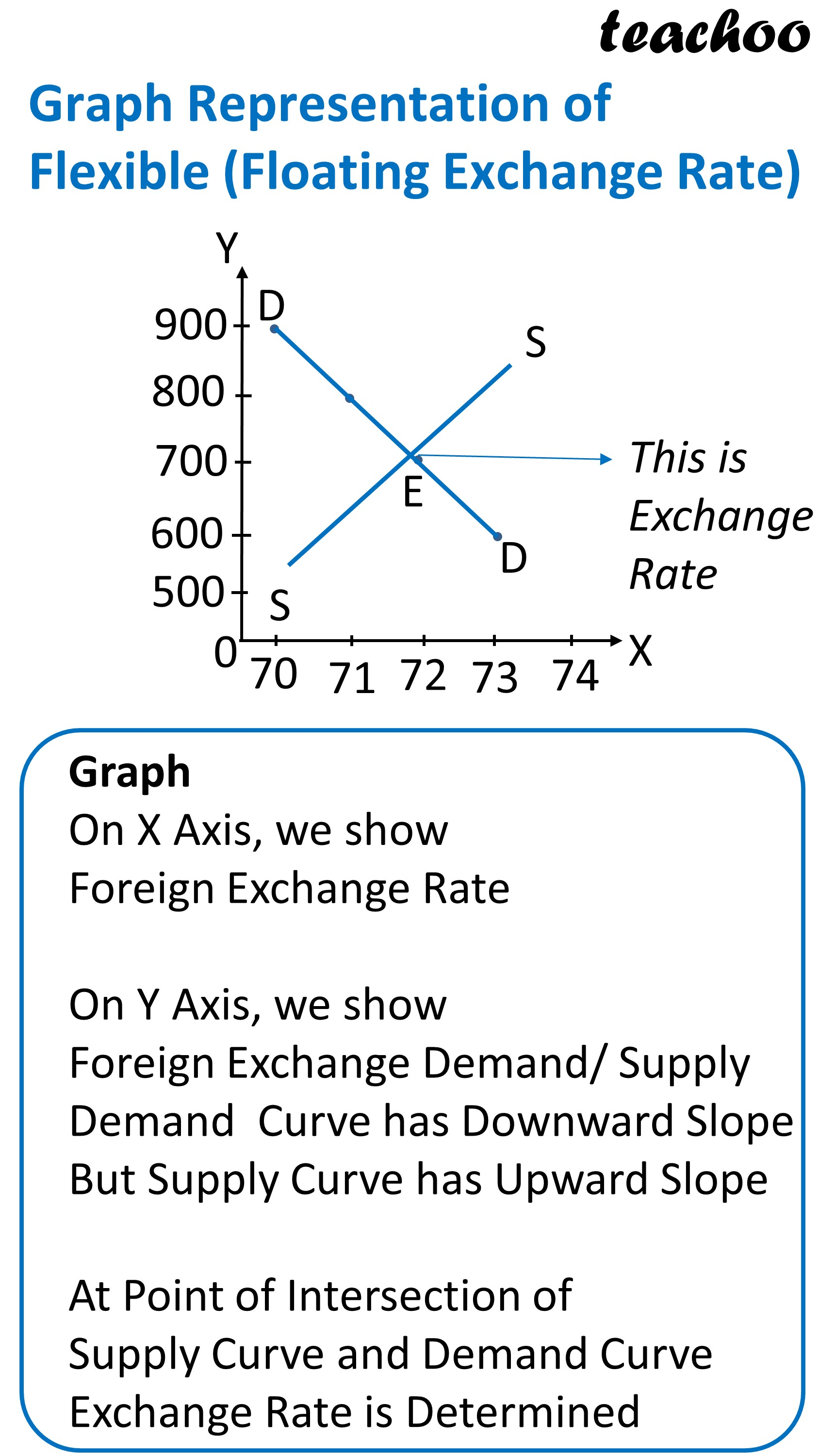Chapter 6 Part 1 - Foreign Exchange Rates

Economics Class 12
Macroeconomics

It is a system where

Exchange Rate is determined by Market Forces of Demand and Supply

In this case, Central Bank does not intervene (interfere) in determining exchange rate

## How is Foreign Exchange Rate Determined?

It is Determined at a Point where Demand and Supply Curve Intersect each other#### Note

In Flexible Exchange Rate,

There is appreciation and depreciation of domestic currency

### NCERT Questions

No questions in this part

### Other Books

#### Question 1

In the following questions, select the correct answers:

Flexible exchange rate system is also known as :

1. Pegged Exchange rate system
2. Dirty Floating
3. Floating exchange rate system
4. Both B and C

Learn in your speed, with individual attention - Teachoo Maths 1-on-1 Class

### Transcript

What is Flexible or Floating Exchange Rate Year 2020 2021 2022 Exchange Rate 70 75 80 In this case, Exchange Rate is determined by forces of Demand and Supply Demand of Foreign Exchange Supply of Foreign Exchange More the Exchange Rate Less the Foreign Exchange Demand (it is Inversely Proportional) More the Exchange Rate More the Foreign Exchange Supply (it is Directly Proportional) Schedule Representation of Flexible (Floating Exchange Rate) Foreign Exchange Rate 70 71 72 73 Foreign Exchange Demand 900 800 700 600 Foreign Exchange Supply 500 600 700 800 This Decreases with Increase in Rate This Increases with Increase in Rate Here Demand=Supply This is how Foreign Exchange Rate Determined Graph Representation of Flexible (Floating Exchange Rate) This is Exchange Rate Graph On X Axis, we show Foreign Exchange Rate On Y Axis, we show Foreign Exchange Demand/ Supply Demand Curve has Downward Slope But Supply Curve has Upward Slope At Point of Intersection of Supply Curve and Demand Curve Exchange Rate is Determined